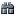### Class IndexQuicksearch
###### Option `write_converters`¶ ↑

Specifies converters to be used in generating fields. See Write Converters

Default value:

```CSV::DEFAULT_OPTIONS.fetch(:write_converters) # => nil
```

With no write converter:

```str = CSV.generate_line(["\na\n", "\tb\t", " c "])
str # => "\"\na\n\",\tb\t, c \n"
```

With a write converter:

```strip_converter = proc {|field| field.strip }
str = CSV.generate_line(["\na\n", "\tb\t", " c "], write_converters: strip_converter)
str # => "a,b,c\n"
```

With two write converters (called in order):

```upcase_converter = proc {|field| field.upcase }
downcase_converter = proc {|field| field.downcase }
write_converters = [upcase_converter, downcase_converter]
str = CSV.generate_line(['a', 'b', 'c'], write_converters: write_converters)
str # => "a,b,c\n"
```

Raises an exception if the converter returns a value that is neither `nil` nor String-convertible:
```bad_converter = proc {|field| BasicObject.new }